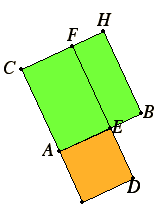# Proposition 30

To cut a given finite straight line in extreme and mean ratio.

Let AB be the given finite straight line.It is required to cut AB in extreme and mean ratio.

Describe the square BC on AB. Apply the parallelogram CD to AC equal to the sum of BC and the figure AD similar to BC.

Now BC is a square, therefore AD is also a square.

And, since BC equals CD, subtract CE from each, therefore the remainder BF equals the remainder AD.

VI.14
But it is also equiangular with it, therefore in BF and AD the sides about the equal angles are reciprocally proportional. Therefore FE is to ED as AE is to EB.

But FE equals AB, and ED equals AE.

Therefore AB is to AE as AE is to EB.

And AB is greater than AE, therefore AE is also greater than EB.

Therefore the straight line AB has been cut in extreme and mean ratio at E, and the greater segment of it is AE.

Q.E.F.

## Guide

The construction given here cuts a line into two parts A and B so that (A + B):B = B : A. By proposition VI.17, that condition is equivalent to making the rectangle A + B by A equal the square B by B, and that is the construction of proposition II.11.

#### Use of this proposition

This construction is used in XIII.17 to construct a pentagonal face of a regular dodecahedron.